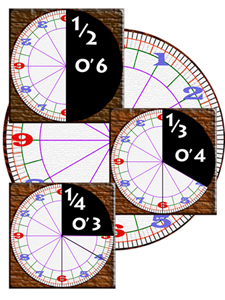What about decimals?

Any number base can have expressions such as 0.5 etc - the decimal version of a half in base ten. One-half is five tenths, hence 0.5, but it is also six twelfths, or 0.6 in base twelve. We need to distinguish decimals from dozenals, so we can either use the star and point, as in *0.6, to mean a half in base twelve, or use a different kind of "dozenal point". Following suggestions made on the Dozenal Forum we have decided to use the symbol ' as a dozenal point, and write 0'6 to mean a half in base twelve. (There have been other suggestions, which you can read about elsewhere on this site).

Because twelve can be divided exactly by three the fraction one-third is simpler in base twelve; as four twelfths is is simply 0'4. A quarter becomes 0'3, shorter than the decimal 0.25 (twenty-five hundredths). Then a sixth is 0'2, an eighth is 0'16, a sixth 0'2, a ninth 0'14 and a twelfth 0'1.

In exchange for these simpler forms we lose the fifth and tenth as simple expressions as five does not divide twelve exactly. Just as a tird and a sixth have repeating patterns in decimals, so do the fifth and tenth in dozenals.

(to be continued)